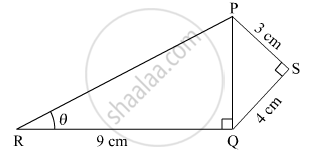# In Figure 1, PS = 3 cm, QS = 4 cm, ∠PRQ = θ, ∠PSQ = 90°, PQ ⊥ RQ and RQ = 9 cm. Evaluate tan θ. - Mathematics

SumIn Figure 1, PS = 3 cm, QS = 4 cm, ∠PRQ = θ, ∠PSQ = 90°, PQ ⊥ RQ and RQ = 9 cm. Evaluate tan θ.

#### Solution

Given,
PS = 3 cm, QS = 4 cm, RQ = 9cm

In In ΔPSQ,
PQ2 =PS2 + OS2

PQ2 = 32 +42 = 25

⇒ PQ = 5 cm

In Δ PQR,

tan θ ="PQ"/"RQ"
⇒ tan θ =5/9

Is there an error in this question or solution?
2018-2019 (March) All India (Set 2)

Share